﻿ Use of Correlation Coefficient and Quartiles of Auxiliary Variable for Improved Estimation of Population VarianceAmerican Journal of Operational Research

p-ISSN: 2324-6537    e-ISSN: 2324-6545

2016;  6(2): 33-39

doi:10.5923/j.ajor.20160602.01### Use of Correlation Coefficient and Quartiles of Auxiliary Variable for Improved Estimation of Population Variance

Subhash Kumar Yadav1, S. S. Mishra1, Alok Kumar Shukla2

1Department of Mathematics and Statistics (A Centre of Excellence), Dr. RML Avadh University, Faizabad, U.P., India

2Department of Statistics, D.A.V College, Kanpur, U.P., India

Correspondence to: S. S. Mishra, Department of Mathematics and Statistics (A Centre of Excellence), Dr. RML Avadh University, Faizabad, U.P., India.
 Email: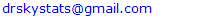Abstract

The present paper deals with the estimation of population variance using correlation coefficient and quartiles of an auxiliary variable under simple random sampling scheme. Up to the first order of the approximation, the bias and the mean square error of the proposed estimator have been obtained. The optimum value of the characterizing scalar kappa has been obtained and for this optimum value of kappa, the minimum mean square error of the proposed estimator has also been obtained up to the first order of approximation. A comparison of the proposed estimator has been made with existing estimators of population variance under simple random sampling scheme. An empirical study is also carried out to justify the theoretical findings. An improvement over existing estimators has been shown in the sense of having lesser mean square error.

Keywords: Ratio estimator, Quartiles, Bias, Mean squared error, Efficiency

Cite this paper: Subhash Kumar Yadav, S. S. Mishra, Alok Kumar Shukla, Use of Correlation Coefficient and Quartiles of Auxiliary Variable for Improved Estimation of Population Variance, American Journal of Operational Research, Vol. 6 No. 2, 2016, pp. 33-39. doi: 10.5923/j.ajor.20160602.01.

### 1. Introduction

In survey sampling, auxiliary information is used for enhancing the efficiency of the estimate of the parameters of the population for the characteristic under study. Auxiliary information is supplied by the auxiliary variable which is highly positively or negatively correlated with the main variable under study. Ratio type estimators are used when the variables x and y are positively correlated and the line of regression of y on x passes through origin, while the product type estimators are used when x and y are negatively correlated to each other otherwise regression estimators are used. In the present study, we are dealing with only the positive correlation.
Let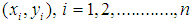be the n pair of observations for the auxiliary and study variables, respectively from the population of size N using simple random sampling without replacement. Let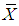and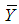be the population means of auxiliary and study variables respectively and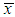and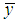be the respective sample means.
Following are the notations which have been used in this manuscript and are already being discussed by Subramani and Kumarpandiyan (2015) as: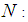Size of the population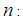Size of the sample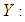Study variable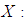Auxiliary variable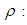Correlation coefficient between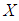and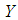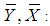Population means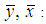Sample means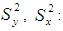Population variances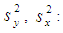Sample variances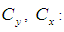Coefficient of variations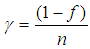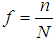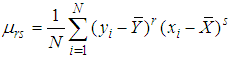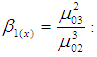Skewness of the auxiliary variable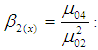Kurtosis of the auxiliary variable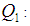First quartile of the auxiliary variable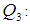Third quartile of the auxiliary variable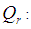Inter quartile range of the auxiliary variable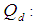Semi quartile range of the auxiliary variable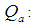Semi quartile average of the auxiliary variable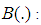Bias of the estimator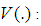Variance of the estimator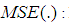Mean squared error of the estimator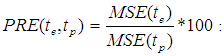Percentage relative efficiency of the estimator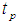over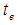The appropriate estimator of the population variance is the sample variance defined as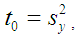(1.1)
which is an unbiased estimator of the population variance and its variance up to the first order of approximation is given as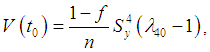(1.2)
where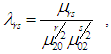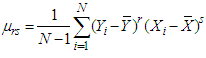and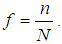Isaki (1983) utilizing the auxiliary information, proposed the following classical ratio estimator for the population variance as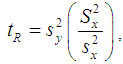(1.3)
where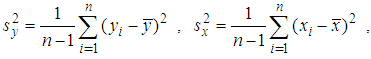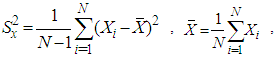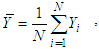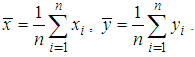,
The expressions for the Bias and Mean Square Error (MSE) of the estimator in (1.3) up to the first order of approximation respectively are as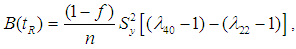(1.4)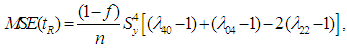(1.5)
(Vide Kendall and Stuart (1977))
In the series of improvement, many authors have proposed different estimators of population variance utilizing the parameters of the auxiliary variable. Following table-1 which was also given by Subramani and Kumarpandiyan (2012) represents different estimators along with their bias, mean square error and constant.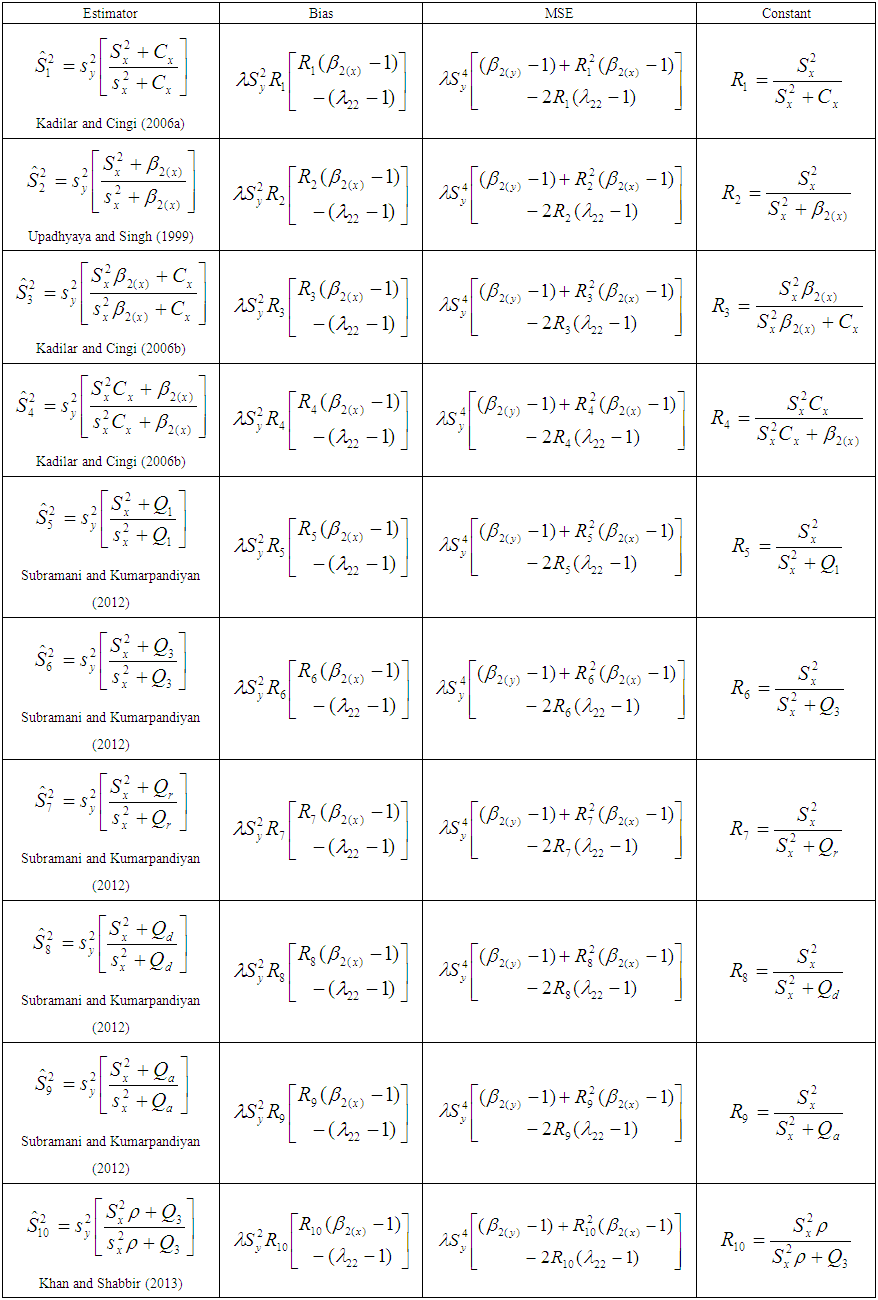Table 1. Bias, MSE and Constants of different estimators
Where symbols have their usual meanings.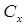is the coefficient of variation of auxiliary variable,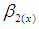is the coefficient of kurtosis,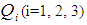are the quartiles of the auxiliary variable.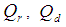and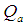are the functions of quartiles defined by,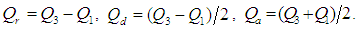Thus, in general the mean square errors of the estimators given in above table may be written as,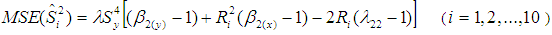(1.6)
The latest references in the series of improvement can be made of Shukla et al. (2015) and Yadav et al. (2015a, 2015b, 2016).

### 2. Proposed Estimator

Motivated by Prasad (1989) and Khan and Shabbir (2013), we propose an efficient ratio estimator of population variance as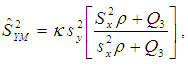(2.1)
where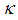is a suitable constant to be determined such that the mean square error of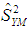is minimum.
In order to study the large sample properties of the proposed estimator,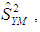we define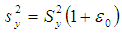and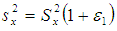with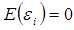for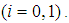In case of simple random sampling without replacement, ignoring finite population correction term, the following expectations could be obtained either directly or by the method due to Kendall and Stuart (1977) as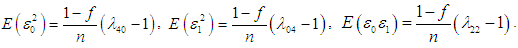Expressing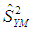in terms of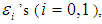we have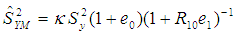After simplifying and retaining terms up to the first order of approximation, we have: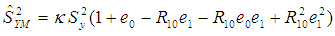Subtracting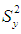on both the sides, we obtain,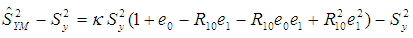(2.2)
Taking expectation on both sides of (2.2), we have the bias of proposed estimator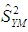as: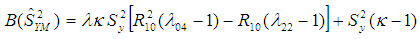(2.3)
where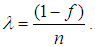The mean squared error of the proposed estimator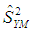is obtained by squaring both sides of (2.2), simplifying and taking expectation on both sides, up to the first order of approximation as,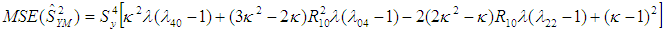(2.4)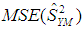is minimum for,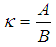(2.5)
where,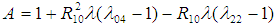and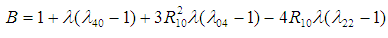The minimum MSE of the estimator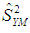for this optimum value of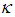is: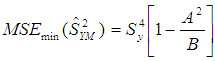(2.6)
Here, we pass an important remark that the value of the unknown parameters involved in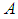and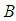can be obtained from the previous surveys or the experience gathered in due course of time, for instance, vide Murthy (1967), Reddy (1973, 1974), Srivenkataramana & Tracy (1980), Singh & Vishwakarma (2008), Singh & Kumar (2008) and Singh & Karpe (2010). If the unknown parameters inandare replaced by their estimates, then we obtain the same minimum mean squared error as in (2.6).

### 3. Efficiency Comparison

From (2.6) and (1.2), we have: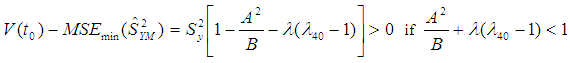(3.1)
From (2.6) and (1.5), we have: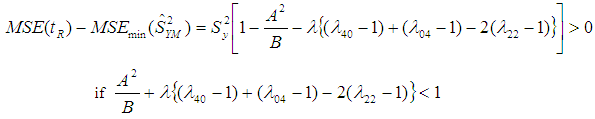(3.2)
From (2.5) and (1.6), we have: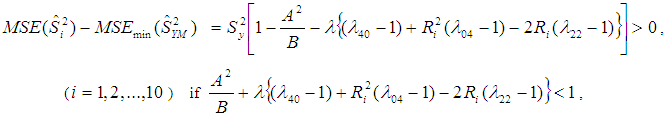(3.3)

### 4. Numerical Illustration

To justify the theoretical findings of different estimators, we have considered the following real populations.
Population-1: Italian bureau for the environment protection-APAT Waste 2004
Y: Total amount (tons) of recyclable-waste collection in Italy in 2003.
X: Total amount (tons) of recyclable-waste collection in Italy in 2002.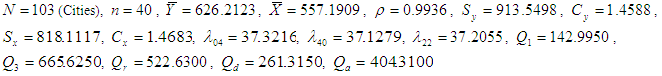Population-2: Italian bureau for the environment protection-APAT Waste 2004
Y: Total amount (tons) of recyclable-waste collection in Italy in 2003.
X: Number of inhabitants in 2003.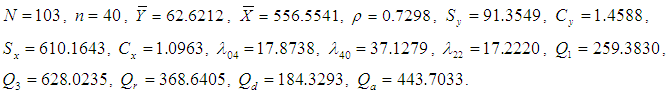Population-3: Murthy (1967)
Y: Output for 80 factories in a region.
X: Fixed capital.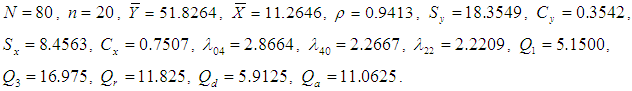Population-4: Singh and Chaudhary (1986)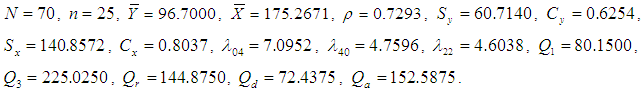### 5. Observation and Conclusions

Table-1 shows the Bias, MSE and Constants for previously existing estimators given by different researchers. Proposed estimator which has been subjected to comparisons with previous estimators nothing but is a ratio estimator. In this paper, we have been able to develop more efficient estimator whose bias is significantly far much less as compared to previous estimators as evidently presented in table-2. Similarly, MSE is also far much less as compared to previous estimators given in the table-2. Moreover, table-3 shows that relative efficiency is comparatively much higher. Thus, we can finally conclude with passing remarks that from theoretical discussions in section-3 and the results in table-2 and 3, we infer that the proposed estimator is much better than the previously existing estimators of population mean in simple random sampling scheme, therefore proposed estimator should be preferred for the estimation of population variance.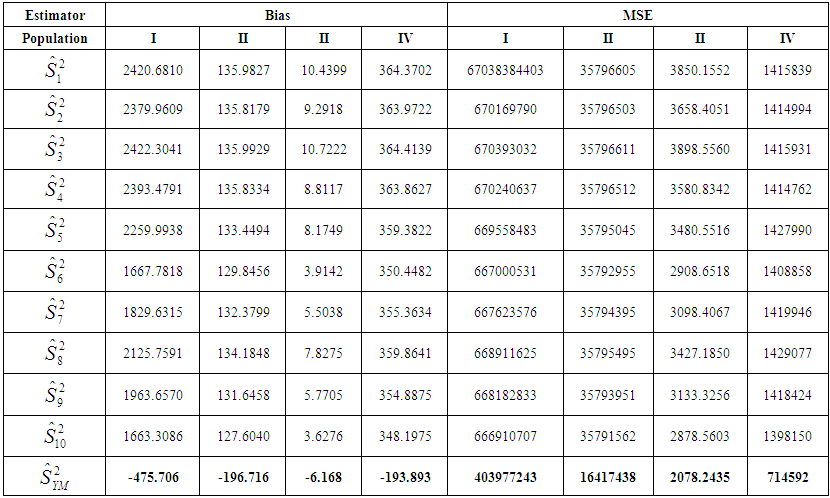Table 2. Bias and Mean square error of different estimators
 Table 3. Percentage Relative Efficiency (PRE) of different estimators with respect to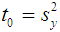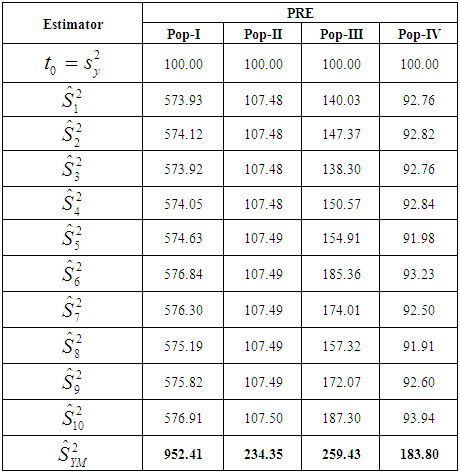### ACKNOWLEDGMENTS

The authors are very much thankful to the editor of American Journal of Operational Research and the anonymous referees for critically examining the manuscript and giving the valuable suggestions to improve the manuscript in present form.

### References

  Isaki, C, T., (1983). Variance estimation using auxiliary information, Journal of American Statistical Association, 78, 117- 123.  Kadilar, C. and Cingi, H., (2006a). Improvement in variance estimation using auxiliary information, Hacettepe Journal of mathematics and Statistics, 35, 111-15.  Kadilar, C. and Cingi, H., (2006b). Ratio estimators for population variance in simple and Stratified sampling, Applied Mathematics and Computation, 173, 1047-1058.  Kendall, M. and Stuart, A., (1977). The advanced theory of statistics, Vol I, Charles Griffin & Co., London.  Khan, M. and Shabbir, J., (2013). A Ratio Type Estimator for the Estimation of Population Variance using Quartiles of an Auxiliary Variable, Journal of Statistics Applications & Probability, 2, 3, 319-325.  Murthy, M. N., (1967). Sampling Theory and Methods, Statistical Publishing Society Calcutta, India.  Prasad, P. (1989). Some improved ratio type estimators of population mean and ratio in finite population sample surveys, Communications in Statistics: Theory and Methods, 18, 379-392.  Reddy, V., (1973). On ratio and product methods of estimation’, Sankhya Serie B 35(3), 307–316.  Reddy, V., (1974). On a transformed ratio method of estimation’, Sankhya Serie C 36, 59–70.  Singh, D. and Chaudhary, F. S., (1986). Theory and analysis of sample survey designs, New-Age International Publisher.  Shukla, A.K, Misra, S., Mishra, S.S. and Yadav, S.K (2015). Optimal Search of Developed Class of Modified Ratio Estimators for Estimation of Population Variance, American Journal of Operational Research, 5, 4, 82-95.  Singh, H. and Karpe, N. (2010). Estimation of mean, ratio and product using auxiliary information in the presence of measurement errors in sample surveys’, Journal of Statistical Theory and Practice 4(1), 111–136.  Singh, H. and Kumar, S., (2008). A general family of estimators of finite population ratio, product and mean using two phase sampling scheme in the presence of non-response’, Journal of Statistical Theory and Practice 2(4), 677–692.  Singh, H. and Vishwakarma, G., (2008). Some families of estimators of variance of stratified random sample mean using auxiliary information, Journal of Statistical Theory and Practice 2(1), 21–43.  Srivenkataramana, T. and Tracy, D. (1980). An alternative to ratio method in sample surveys’, Annals of the Institute of Statistical Mathematics 32, 111–120.  Subramani, J. and Kumarapandiyan, G. (2012). Variance estimation using quartiles and their functions of an auxiliary variable, International Journal of Statistics and Applications, 2, 67-72.  Subramani, J. and Kumarapandiyan, G. (2015). A class of modified ratio estimators for estimation of population variance. Jamsi, 11, 1, 91-114.  Upadhyaya, L.N. and Singh, H.P. (1999). An estimator for population variance that utilizes the kurtosis of an auxiliary variable in sample surveys, Vikram Mathematical Journal, 19, 14-17.  Yadav, S.K., Kadilar, C., Shabbir, J. and Gupta, S. (2015a). Improved Family of Estimators of Population Variance in Simple Random Sampling, Journal of Statistical Theory and Practice, 9, 2, 219-226.  Yadav, S.K., Mishra, S.S., Shukla, A.K. and Tiwari, V. (2015b). Improvement of Estimator for Population Variance using Correlation Coefficient and Quartiles of The Auxiliary Variable, Journal of Statistics Applications and Probability, 4, 2, 259-263.  Yadav, S.K., Misra, S. and Mishra, S.S. (2016). Efficient Estimator for Population Variance Using Auxiliary Variable, American Journal of Operational Research, 6, 1, 9-15.  http://www.osservatorionalerifiuti.it/ElencoDocPub.asp?A_TipoDoc=6.